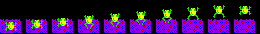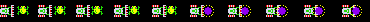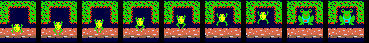# Frogger Tutorial part 3

System Message: WARNING/2 (<string>, line 5)

Explicit markup ends without a blank line; unexpected unindent.

# The frog

So far the game code has avoided any state variables - all the animation has been hung off the global time counter t. But the frog requires that the game keeps track of some state in variables. These are the position of the frog in X and Y, and a couple more variables to keep track of the frog's animation:

```static int frogx, frogy;    // screen position
static int leaping;         // 0 means not leaping, 1-8 animates the leap
static int frogdir;         // while leaping, which direction is the leap?
static int frogface;        // which way is the frog facing, as a sprite ROT field
static int dying;           // 0 means not dying, 1-64 animation counter
```

Two of these variables (leaping and dying) do double work: they are flags and counters. When leaping is zero it means that the frog is sitting still, when it is non-zero a leap is in progress, and it counts the animation cycles until the leap is complete.

Because it is a good idea to initialize all these variables in one place, next step is to make a function frog_start():

```void frog_start()
{
frogx = 120;
frogy = 232;
leaping = 0;
frogdir = 0;
frogface = 0;
dying = 0;
}
```

and call it in from our setup() function.

The code that draws the frog uses these variables:

```  // The frog himself, or his death animation
byte frogspr = GD.spr; // record which sprite slot frog got, for collision check below

if (!dying) {
static byte frog_anim[] = {2, 1, 0, 0, 2};
sprite(frogx, frogy, frog_anim[leaping / 2], frogface);
} else {
static byte die_anim[] = {31, 32, 33, 30};
sprite(frogx, frogy, die_anim[dying / 16], frogface);
}
```

If the frog is alive and leaping, the frog is drawn using frames 2, 1 and 0. If the frog is dying, then frames 31, 32, 33, and 30 are used. Either way, the code follows the same pattern: an array of animation frames (frog_anim or die_anim) is indexed by the animation variable. To slow down the animation to the correct speed, the variables are divided by 4 and 16 respectively. The range of the animation variable, the size of the animation array and the divider are all tied together.

To illustrate, here is how the leaping counter's value from 0-8 controls the animation frame:

 leaping 0 1 2 3 4 5 6 7 8 leaping / 2 0 0 1 1 2 2 3 3 4 frog_anim[leaping / 2] 2 2 1 1 0 0 0 0 2The last piece of code handles the actual business of reading the buttons and setting the frog's variables:

```  // player control.  If button pressed, start the 'leaping' counter
if (!dying && (leaping == 0) && con) {
frogdir = con;
leaping = 1;
score += 10;
} else if (leaping > 0) {
if (leaping <= 8) {
if (frogdir == CONTROL_LEFT) {
frogx -= 2;
frogface = 3;
} if (frogdir == CONTROL_RIGHT) {
frogx += 2;
frogface = 5;
} if (frogdir == CONTROL_UP) {
frogy -= 2;
frogface = 0;
} if (frogdir == CONTROL_DOWN) {
frogy += 2;
frogface = 6;
}
leaping++;
} else {
leaping = 0;
}
}
```

# Dying

The code to handle the dying animation checks the dying state variable, and if it is non-zero, increments it. When dying reaches 64, the animation is over and the game decides whether the player has another life remaining.

```  if (dying) {
if (++dying == 64) {
if (--lives == 0) {
game_start();
level_start();
}
frog_start();
}
}
else if (frogx < 8 || frogx > 224) {
dying = 1;
}
```

When the frog touches a screen-edge, it dies.It's sad, but the frog dies when it touches a car. To make this happen, the game detects when the frog touches abother sprite, then sets the dying variable.

```  GD.waitvblank();
byte touching = (GD.rd(COLLISION + frogspr) != 0xff);
```

These two lines of code are enough to detect a collision between the frog and other sprite. The first line waits for the whole screen to display, and the second line reads the Gameduino's collision detection RAM to find out if the frog sprite is touching any other sprite (see example collision).

```  else if (frogy >= 136) {    // road section
// if touching something, frog dies
if (touching)
dying = 1;
}
```

This test follows on from the screen-edge test above. If we're in the road section (which starts at Y-coordinate 136), if an a car sprite touches the frog, it kills it.

# River section: riding the logs

The river's logic is different. When the frog is touching another sprite (a log or a turtle) then it is safe. When it's not touching something, the frog must be sitting in the river, so it drowns and dies.

But the river is different in another way: the frog moves sideways with whatever it is standing on. This needs a small amount of cunning to figure out the river's sideways speed, then move the frog by that amount:

```  else if (frogy > 40) {      // river section
if (!leaping) {
// if touching something, frog is safe
if (touching) {
// move frog according to lane speed
int oldx = riverat(frogy, t - 1);
int newx = riverat(frogy, t);
int river_velocity = newx - oldx;
frogx += river_velocity;
} else {
dying = 1;
}
}
}
else
```

This is why the riverat() function exists: it lets us figure out how much to move the frog. When the frog is standing on something, the code calls riverat() twice, once with the time one tick in the past (t - 1), and once with the current time (t). The difference of these two positions is the river speed (in pixels per tick). Adding this to the frog's X-coordinate moves it at the same speed as the log or turtle. If the frog stays still too long, it will be carried off the edge of the screen and the screen-edge logic above will kill it.

# The riverbank: winningWhen the frog leaps up past the river and onto the far bank, two things can happen. If the frog lands in an empty home, that home gets filled with sprite 63 (http://gameduino.com/results/ceb9b4e1/) and the player moves back to the start. Otherwise, the frog dies.

The array homes[] holds the X-coordinate of each of the home slots, and the array done[] keeps track of which slots have been filled. The code decides if the leaping frog is within 4 pixels of home i with the expression abs(homes[i] - frogx) < 4, and sets the flag landed if so. If landed is false, then the frog did not end up in one of the homes, and it dies:

```  {                      // riverbank section
if (!leaping) {
byte landed = 0;
for (byte i = 0; i < 5; i ++) {
if (!done[i] && abs(homes[i] - frogx) < 4) {
done[i] = 1;
landed = 1;
score += 10;
}
}
if (landed) {
if (done && done && done && done && done)
level_start();
frog_start();
} else // if frog did not land in a home, die!
dying = 1;
}
}
```

# Sound

The original game's sound is very simple - there is only one note playing at a time. The sound() functions looks at state variables - leaping and dying again - and figures out what note to play. It uses a table of note frequencies (midifreq) so it can use MIDI note numbers instead of raw frequencies. The leaping sound is made of pairs of notes one octave (12 midi notes) apart, played in quick succession, a common effect in vintage video games.

To get that authentic vintage game sound, the function squarewave simulates a square-wave using the math described in the Gameduino cookbook.

```// midi frequency table
static PROGMEM prog_uint16_t midifreq = {
32,34,36,38,41,43,46,48,51,55,58,61,65,69,73,77,82,87,92,97,103,110,116,123,130,138,146,155,164,174,184,195,207,220,233,246,261,277,293,311,329,349,369,391,415,440,466,493,523,554,587,622,659,698,739,783,830,880,932,987,1046,1108,1174,1244,1318,1396,1479,1567,1661,1760,1864,1975,2093,2217,2349,2489,2637,2793,2959,3135,3322,3520,3729,3951,4186,4434,4698,4978,5274,5587,5919,6271,6644,7040,7458,7902,8372,8869,9397,9956,10548,11175,11839,12543,13289,14080,14917,15804,16744,17739,18794,19912,21096,22350,23679,25087,26579,28160,29834,31608,33488,35479,37589,39824,42192,44701,47359,50175
};

static void squarewave(uint16_t freq, byte amp)
{
GD.voice(0, 0, freq,     amp,    amp);
GD.voice(1, 0, 3 * freq, amp/3,  amp/3);
GD.voice(2, 0, 5 * freq, amp/5,  amp/5);
GD.voice(3, 0, 7 * freq, amp/7,  amp/7);
GD.voice(4, 0, 9 * freq, amp/9,  amp/9);
GD.voice(5, 0, 11 * freq, amp/11,  amp/11);
}

static void sound()
{
byte note;

if (dying) {
note = 84 - (dying / 2);
squarewave(MIDI(note), 100);
} else if (leaping) {
if (leaping & 1)
note = 60 + leaping;
else
note = 72 + leaping;
squarewave(MIDI(note), 100);
} else {
squarewave(0, 0);  // silence
}
}
```

# Moving the action to the center of the screen

Up until now, the game has appeared at the left edge of the screen, and sprites have overlapped screen edges. This is not a polished look.

The first step is to move all the background graphics to the center of the screen - 11 characters to the right. Because all background graphics addresses use the atxy() function, that's the perfect spot to make this change, just by adding 11 to the X-coordinate:

```static uint16_t atxy(byte x, byte y)
{
return RAM_PIC + 64 * y + (x + 11);
}
```

Similarly, all sprites are drawn with the sprite() function, so it also shifts everything to the right:

```static void sprite(byte x, byte y, byte anim, byte rot = 0)
{
draw_sprite(x + 80, y, anim, rot);
}
```

Lastly, the sprites need to disappear at the screen edges, instead of poking out at the sides. The easiest way of doing this is to use two columns of solid black square sprites to cover up the screen edges. This code paints the columns using sprite numbers 255 down. These "curtain" sprites are in high slots and so cover up the actual game sprites.

```  byte i = 255;
for (int y = 0; y < 256; y += 16) {
GD.sprite(i--, 72, y, 63, 0);
GD.sprite(i--, 72 + 240, y, 63, 0);
GD.sprite(i--, 72 + 256, y, 63, 0);
}
```

# The finished sketch

The finished game, available as a sketch here: frogger.zip.

Some data on the game:

• about 15K total used flash from the Arduino's 32K
• about 350 lines of code in the frogger.pde sketch